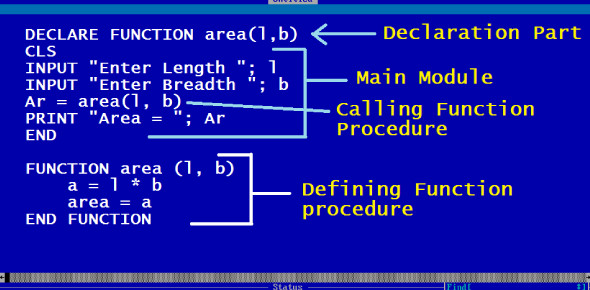# QBasic Programming Quiz Questions And Answers

15 Questions | Attempts: 16399
ShareSettingsAre you ready to attempt these QBasic programming quiz questions and answers? Yes, this is a quiz which is about QBasic programming. Do you know a lot about this? This is the time to prove your understanding of it. Try to answer these given questions. After the quiz, if you somehow manage to get a score above 70%, then you can consider yourself to have passed this test. Let's go now!

• 1.
What will the following code produce? PRINT "Afrikom Computer School"
• A.

A paper printed with Afrikom Computer School

• B.

A screen printed with Afrikom Computer School

• C.

None of the above

• D.

An error indicated on the screen

• 2.
If the English language follows formats and rules on grammar, what does QBasic follow as a counterpart?
• A.

Strings

• B.

Syntax

• C.

Grammar

• D.

All of the above

• 3.
"Name\$" is known as a
• A.

Stringer

• B.

Constant

• C.

Variable

• D.

Input driver

• 4.
Text that you wish to appear on the screen while your program runs must appear inside of ---------------------
• A.

Slashes

• B.

Brackets

• C.

Quotes

• D.

Braces

• 5.
What must you do if "Syntax Error" appears?
• A.

Check for flaws, edit, and rerun. It may be a programmer's error.

• B.

Close the program

• C.

Delete it

• D.

None of the above

• 6.
A computer program is simply:
• A.

An exercise of the brain

• B.

A set of instructions to tell a computer how to perform a particular task

• C.

Pairs of computer system

• D.

None of the above

• 7.
An algorithm is:
• A.

The output of the instruction to the computer.

• B.

The required data to be processed.

• C.

A finite sequence (or series) of precise instructions for solving a problem.

• D.

Problem definition

• 8.
The following symbols are used in flowcharting, except:
• A.

The terminal symbol

• B.

The process symbol

• C.

The input/output symbol

• D.

The cross symbol

• 9.
The following are types of errors in programming, except:
• A.

Grammar error

• B.

Syntax error

• C.

Logic error

• D.

Compile error

• 10.
What will be the output of the following code? 10 REM EXAMPLE OF HOW A COMMA 20 REM AFFECTS THE OUTPUT 30 REM PRINT STATEMENT 40 PRINT  “4 + 7 = ”, 50 PRINT  4 + 7 60 PRINT END
• A.

4 + 7

• B.

4 + 7 = 11

• C.

11

• D.

All of the above

• 11.
The CLS statement:
• A.

Is to instruct the user to enter data

• B.

To enter data

• C.

Is used for clearing the screen.

• D.

All of the above

• 12.
BASIC is a typical example of an interpreted language.
• A.

True

• B.

False

• 13.
What is the acronym for BASIC?
• A.

Beginner Action- Purpose Symbolic Instruction Code

• B.

Basic Algorithm Sequence Instruction of Computer

• C.

Beginner's All-Purpose Symbolic Instruction Code

• D.

None of the above

• 14.
The following arithmetic operations are carried out in BASIC except
• A.

^

• B.

*

• C.

+

• D.

X

• 15.
This expression (a+b) 3 can be written in BASIC as follows:
• A.

(a+b)^3

• B.

(a+b)*(a+b)*(a+b)

• C.

Both as in A and B

• D.

(a+b)x(a+b)x(a+b)

## Related TopicsBack to top
×

Wait!
Here's an interesting quiz for you.